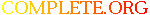Complete.Org: Mailing Lists: Archives: freeciv-ai: May 2002: [freeciv-ai] Re: Amortize# [freeciv-ai] Re: Amortize

[Top] [All Lists]

 To: Subject: [freeciv-ai] Re: Amortize From: "Per I. Mathisen" Date: Wed, 22 May 2002 20:46:24 +0200 (MEST)

```On Wed, 22 May 2002, Raimar Falke wrote:
>  - delay: number of turns that you have to wait till the action will
>  produce something.
>  - surplus(turn) or (s(t) for short):
>     - t>=0
>     - t is relative shifted by current_turn+delay. So s(0) is really
>     s(current_turn+delay).
>     - may be < 0
>  - constant surplus: s(t)=const for all t (simple terrain improvements
>  have a constant surplus)
>  - non-constant surplus: example is the founding of a new city. The
>  surplus depends on the city size and this size isn't fixed.
>  - discrete surplus: s(t) is only defined for integer values of t
>  - decay function: d(t)
>    - d(0)=1
>    - d(t)=0 if t->inf
>    - d(t) is monotonic
>    - example d(t)=0.5^t
>
> Problem 1: we want to decide weither an action A1 which has a delay of
> delay_a1 and a surplus_a1 should be taken or action A2.
>
> First solution: draw up the s(t) and calculate the area under the
> curve.
>
> Problem 1': the area is in most cases infinite.

No, only until endyear and/or spacerace arrival. Games with higher (end
year - current year) have smaller area (if I understood your definition
correctly), and the area shrinks for every turn.

> Problem 2: we want to punish long delays.
>
> Solution 2.1: we multiply the surplus with a decay function. So larger
> delay give smaller overall surplus.
>
> Solution 2.2: _if_ we had finite areas we don't need to care about the
> delay since it will just reduce the area.

Ah, but some improvements (notably cities) give you exponential growth,
and you cannot know the exponential rate, because that is largely decided
by the decisions of the enemy.

I think a decay function is necessary, but the decay rate should be
decided by our evaluation the possibilities for exponential growth, which
in turn largely hinges on our evaluation of the enemy (and endyear and
spacerace).

> Solution 2.1 is the one used in current code. As d(t) (23/24)^t is
> used. This means a decay rate of 1/24 ~= 4.16%. So this decay function
> has a halflife of ln(2)/(1/24)=ln(2)*24 ~= 16.635 turns.

Interesting.

> Note that the current code can only handle constant surplus. If there
> is a non-constant surplus you can either use a symbolic integration
> (hard to very hard) or a numberic integration (also not easy, note
> that the time horizon is unlimited).

We might want to introduce the AI to barriers. Let's say we want to invade
a given enemy in 20 turns. Then we try to get stuff ready in that time
horizon, because we want our military units to be ready, and our
evaluations and needs will probably be turned upside down then anyway,
finished.

This is particularily useful when we have AI diplomacy. Then we can use
periods of peace to expand, and stop building new cities and peaceful
buildings in time for war (when we will probably have to crashbuy lots of
military hardware anyway).

Yours,
Per

"As Israeli forces pursued militants, civilians
continued getting in the way and dying as a
result." -- New York Times, April 21

```